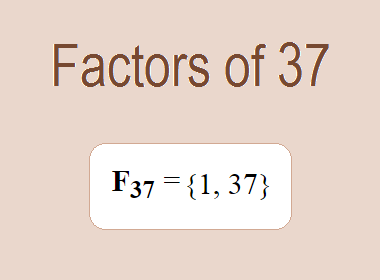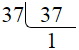# Factors of 37The factors of 37 are 1 and 37 i.e. F37 = {1, 37}. The factors of 37 are all the numbers that can divide 37 without leaving a remainder. 37 is a prime number, so it is divisible by 1 and 37 only.

We can check if these numbers are factors of 37 by dividing 37 by each of them. If the result is a whole number, then the number is a factor of 37. Let's do this for each of the numbers listed above:

·        1 is a factor of 37 because 37 divided by 1 is 37.

·        37 is a factor of 37 because 37 divided by 37 is 1.

## Properties of the Factors of 37

The factors of 37 have some interesting properties. One of the properties is that the sum of the factors of 37 is equal to 38. We can see this by adding all the factors of 37 together:

1 + 37 = 38

Another property of the factors of 37 is that the only prime factor of 37 is 37 itself.

********************

********************

## Applications of the Factors of 37

The factors of 37 have several applications in mathematics. One of the applications is in finding the highest common factor (HCF) of two or more numbers. The HCF is the largest factor that two or more numbers have in common. For example, to find the HCF of 37 and 74, we need to find the factors of both numbers and identify the largest factor they have in common. The factors of 37 are 1, and 37. The factors of 74 are 1, 2, 37, and 74. The largest factor that they have in common is 37. Therefore, the HCF of 37 and 74 is 37.

Another application of the factors of 37 is in prime factorization. Prime factorization is the process of expressing a number as the product of its prime factors. The prime factor of 37 is 37 since it is only the prime number that can divide 37 without leaving a remainder. Therefore, we can express 37 as:

37 = 37

We can do prime factorization by division method as given below,37 = 37

Since 37 is a prime number, there is no factor tree of 37.

## Conclusion

The factors of 37 are the numbers that can divide 37 without leaving a remainder. The factors of 37 are 1, and 37. The factors of 37 have some interesting properties, such as having a sum of 38. The factors of 37 have several applications in mathematics, such as finding the highest common factor and prime factorization.